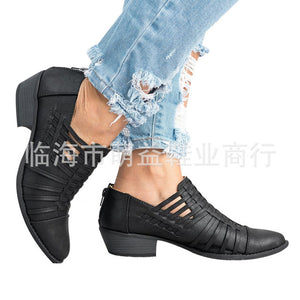\$10 OFF \$89 【CODE: 10】
\$18 OFF \$139 【CODE: 18】
\$25 OFF \$189 【CODE: 25】# Cute Comfortable Women Leather Booties

• \$49.99 USD
Unit price per
• Save \$50.00 USD

Size Note：

Choose your size according to your feet length, and we will be send the corresponding Chinese size. US size 4.5 =CN size 35=22.5 CM, US size 5 =CN size 36=22.8 CM, US size 5.5 =CN size 36=23.0 CM, US size 6 =CN size 37=23.5 CM, US size 6.5 =CN size 38=23.8 CM, US size 7 =CN size 38=24.0 CM, US size 7.5 =CN size 39=24.5 CM, US size 8 =CN size 40=24.8 CM, US size 8.5 =CN size 40=25.0 CM, US size 9 =CN size 41=25.5 CM, US size 9.5 =CN size 42=25.8 CM, US size 10 =CN size 42=26.0 CM, US size 10.5 =CN size 43=26.5 CM,